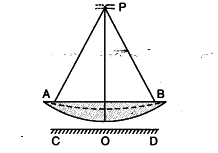# A convex lens is placed over a plane mirror

A convex lens is placed over a plane mirror. A pin is now positioned so that there is no parallax between the pin and its image formed by this lens- mirror combination. How can this observation be used to find the focal length of the convex lens ? Give appropriate reasons in support of your answer

The rays must fall normally on the plane mirror so that the image of the pin coincides with itself.Hence rays, like CA and DB form a parallel beam incident on the lens.
.’. P is the position of the focus of the lens.
Distance OP equals the focal length of the lens